×

### Html & Html5

Introduction to Html

Html5 Grid

Html5 Graphics

Html5 Media

### Python Function

A function is group related statement that performs the specific task.Python provide built-in function like print() etc. you create own function that is called user defined function. We can pass data ,known as parameters into a function.
Function defined in the Python using def keyword.

Syntax of Python :

def function_name(parameters):

statement()

### Calling a function()

To call a function we use the function name and followed by parenthesis()

let's have an example :-

def my_function():

print("hello Python")

my_function()

Once we have create a function and we can call it from another function . To call a function we simply type the function name with appropriate parameters.

### Parameters

Information can be passed to a function known as a parameters.parameters specified after the function name,and inside the parentheses.you can add the more parameter as we want,just separate them with comma.

let's have an example :-

def my_function(fname):

print(fname+ "Hello" )

my_function("Python")

### Output of this Program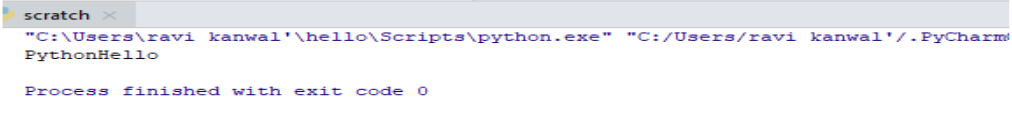So just by calling the function we get our desired output

### Default Parameter Values

If we are call function without any parameter thats is uses the default values .

let's have an example :-

def myFun(x, y=15):

print("x: ", x)

print("y: ", y)

myFun(20)

### Output of this Program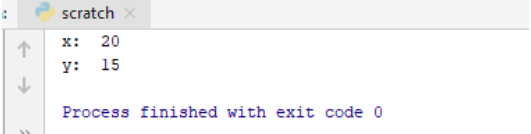here we just assign the value to 'x' but in the output we get the default value of 'y' too.

### Passing a List as a parameters

We can send the all data type parameter to a function such as a (String,Number,list,Dictionary etc..) it will be created same data type in a function.

let's have an example :-

def my_function(food):

for x in food:

print(x)

fruits = ["Banana", "Mango", "Apple"]

my_function(fruits)

### Output of this Program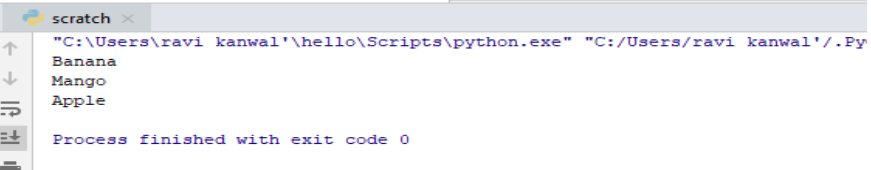here we just get the list in sequence just bvy the calling the function.

### Return value

The return statement with no argument is the same as return none.To let function return we can use return statement.

let's have an example :-

def my_fun(y):

return 9 + y

print(my_fun(3))

print(my_fun(5))

print(my_fun(9))

### Output of this Program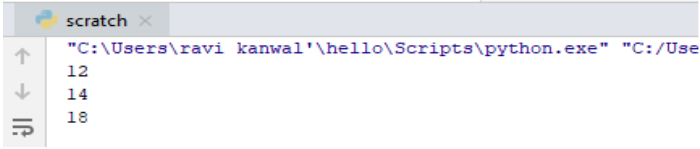So here in return we are getting 9 added to the values we assigned at the time of calling function.

### Recursion

Recursion is process to defining something in terms of itself.

let's have an example :-

def calc_factorial(x):

if x == 1:

return x

else:

return (x * calc_factorial(x-1))

num =5

print("The factorial of", num, "is", calc_factorial(num))

### Output of this Program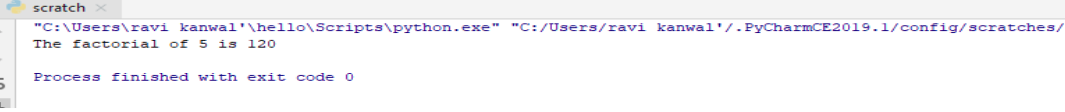So here we get the factorial value of 5.

python training insitute| Best IT Training classes in Gurgaon | python training